Courses

# Current Electricity MCQ Level - 2

## 10 Questions MCQ Test Topic wise Tests for IIT JAM Physics | Current Electricity MCQ Level - 2

Description
This mock test of Current Electricity MCQ Level - 2 for Physics helps you for every Physics entrance exam. This contains 10 Multiple Choice Questions for Physics Current Electricity MCQ Level - 2 (mcq) to study with solutions a complete question bank. The solved questions answers in this Current Electricity MCQ Level - 2 quiz give you a good mix of easy questions and tough questions. Physics students definitely take this Current Electricity MCQ Level - 2 exercise for a better result in the exam. You can find other Current Electricity MCQ Level - 2 extra questions, long questions & short questions for Physics on EduRev as well by searching above.
QUESTION: 1

### A cell of constant emf first connected to a resistance R1 and then connected to resistance R2. If power delivered in both case is same then the internal resistance of the cell is :

Solution: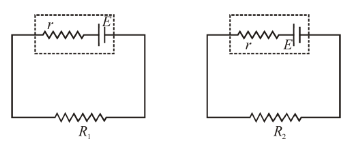We know, Power delivered = i2R  where  i = current and  R = Resistance

Power delivered in both cases is equal then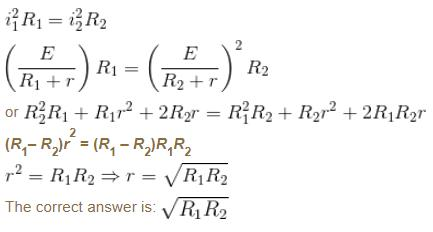QUESTION: 2

### The seals of a galvanometer of resistance 100Ω contains 25 division. It gives a deflection of one division of passing a current of 4 × 10–4A. The resistance in ohm to be added to it, so, that it may become a voltmeter of range 2.5 V is :

Solution:

The deflection of one division of galvanometer is given by the current = 4 × 10–4 A
or the current sensitivity of galvanometer is
= 4 × 10–4 A/div
ig = full scale deflection
= current sensitivity × total number of turns
= 4 × 10–4 × 25 = 10–2 A
Resistance of galvanometer = 100Ω
The value of resistance required,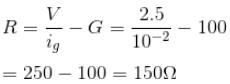QUESTION: 3

### The resistance of the filament of a lamp increase with the increase in temperature. A lamp rated 100W  and 200V  is connected across 220V  power supply. If the voltage drops by 10% then the power of the lamp will be :

Solution:

Let the resistance of the lamp filament be RThen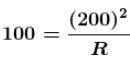when the voltage drops expected power is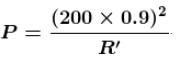Here R'  will be less than R,  because now the rise in temperature will be less. Therefore  P  is more than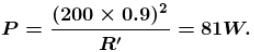But it will not be 90% of earlier value, because fall in temperature is smaller.

The correct answers are: 81 W, between 81 and 90 W

QUESTION: 4

The figure shows the variation of v with I at temperature T1 and T2. (T1 – T2)  is proportional to :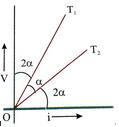Solution:

The Relation between temperature and Resistance is,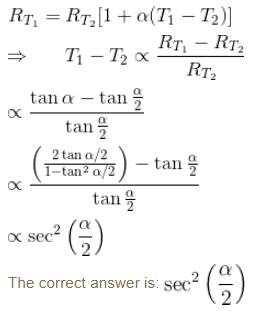QUESTION: 5

The potential difference across the terminal of a battery is 50 V  when 11 A  current is drawn and 60V  when 1A  current is drawn. The emf and the internal resistance of the battery are :

Solution:

For a closed circuit cells supplies a constant current in the circuit.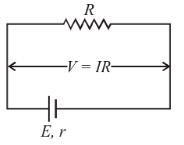Equation of cells E = V + ir
For V = 50 volt
E = 50 + 11r           ...(i)
Similarly for  V = 60V
E = 60 + r      ...(ii)
From Equation (i) and (ii), we get
r = 1Ω
Substituting the value of r  is equation (i), we get
E = 61V
The correct answer is: 61V, 1Ω

QUESTION: 6

Masses of three wire of copper are in ratio 1:3:5, and their length are in ratio 5:3:1. The ratio of their electrical resistance is

Solution:

Ratio of masses of wires
(m1m2m3) = 1 : 3 : 5
Ratio of length of wires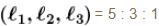Electrical resistance is given by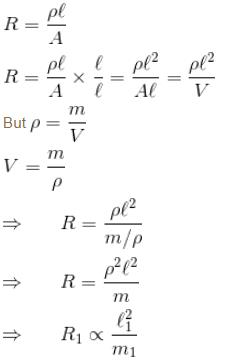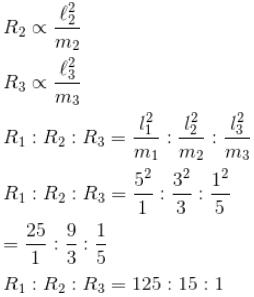The correct answer is: 125 : 15 : 1

QUESTION: 7

When a galvanometer is shorted by resistance S, its current capacity increase n times. If the same galvanometer is shunted by another resistance S', its current capacity will increased by n'  is given by :

Solution:

Using the relation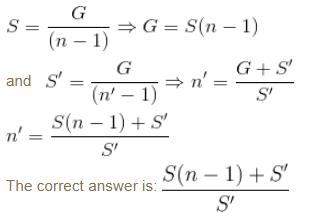QUESTION: 8

A wire of resistance 5Ω is drown out so that its new length is 3 times its original length. What is the resistance of the new wire?

Solution:

Let  L1  is the original length and  L2  is the length after drawn out.
Let L1 l  and  L2 – 3L1 = 3l
Volume is constant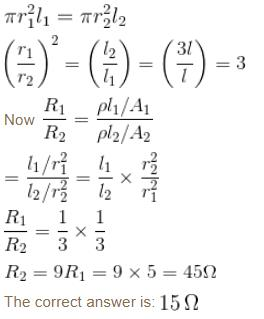QUESTION: 9

The resistance of a wire at 300K is found to be 0.3Ω. If the temperature coefficient of resistance of wire is 1.5 × 10–3 K–1, the temperature at which resistance become 0.6Ω is :

Solution:

Given,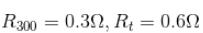T = 300K = 27°C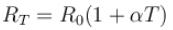Temperature coefficient of resistance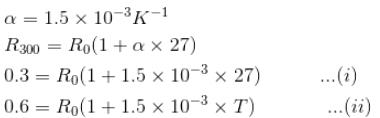Dividing Equation (ii) by equation (i) we get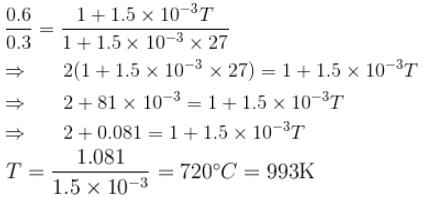QUESTION: 10

An electric immersion heater of 1.08KW is immersed in water. After the water has reached a temperature of 100°C, how much time will be required to produced 100g of steam?

Solution:

L is the latent heat of vaporisation of water, the heat required to produced 1g of steam.

L = 540 cal = 540 × 4. 2 = 2268J.
Energy supplied = 1080 Js–1
Time required to boil 100g  of water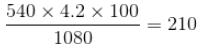The correct answer is: 210 s